# matplotlib.gridspec.GridSpecBase#

class matplotlib.gridspec.GridSpecBase(nrows, ncols, height_ratios=None, width_ratios=None)[source]#

Bases: `object`

A base class of GridSpec that specifies the geometry of the grid that a subplot will be placed.

Parameters:
nrows, ncolsint

The number of rows and columns of the grid.

width_ratiosarray-like of length ncols, optional

Defines the relative widths of the columns. Each column gets a relative width of `width_ratios[i] / sum(width_ratios)`. If not given, all columns will have the same width.

height_ratiosarray-like of length nrows, optional

Defines the relative heights of the rows. Each row gets a relative height of `height_ratios[i] / sum(height_ratios)`. If not given, all rows will have the same height.

get_geometry()[source]#

Return a tuple containing the number of rows and columns in the grid.

get_grid_positions(fig, raw=<deprecated parameter>)[source]#

Return the positions of the grid cells in figure coordinates.

Parameters:
fig`Figure`

The figure the grid should be applied to. The subplot parameters (margins and spacing between subplots) are taken from fig.

rawbool, default: False

If True, the subplot parameters of the figure are not taken into account. The grid spans the range [0, 1] in both directions without margins and there is no space between grid cells. This is used for constrained_layout.

Returns:
bottoms, tops, lefts, rightsarray

The bottom, top, left, right positions of the grid cells in figure coordinates.

get_height_ratios()[source]#

Return the height ratios.

This is None if no height ratios have been set explicitly.

get_subplot_params(figure=None)[source]#
get_width_ratios()[source]#

Return the width ratios.

This is None if no width ratios have been set explicitly.

property ncols#

The number of columns in the grid.

new_subplotspec(loc, rowspan=1, colspan=1)[source]#

Create and return a `SubplotSpec` instance.

Parameters:
loc(int, int)

The position of the subplot in the grid as `(row_index, column_index)`.

rowspan, colspanint, default: 1

The number of rows and columns the subplot should span in the grid.

property nrows#

The number of rows in the grid.

set_height_ratios(height_ratios)[source]#

Set the relative heights of the rows.

height_ratios must be of length nrows. Each row gets a relative height of `height_ratios[i] / sum(height_ratios)`.

set_width_ratios(width_ratios)[source]#

Set the relative widths of the columns.

width_ratios must be of length ncols. Each column gets a relative width of `width_ratios[i] / sum(width_ratios)`.

subplots(*, sharex=False, sharey=False, squeeze=True, subplot_kw=None)[source]#

Add all subplots specified by this `GridSpec` to its parent figure.

See `Figure.subplots` for detailed documentation.

## Examples using `matplotlib.gridspec.GridSpecBase`#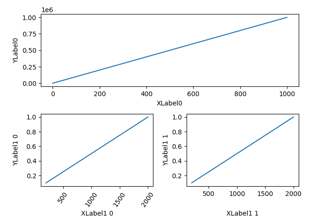Aligning Labels

Aligning Labels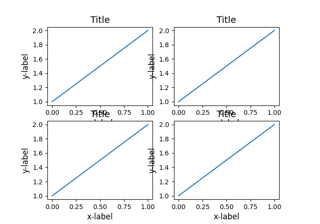Resizing axes with constrained layout

Resizing axes with constrained layout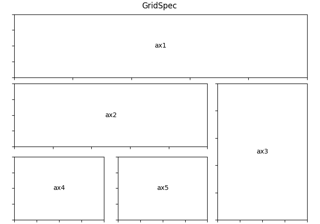Using Gridspec to make multi-column/row subplot layouts

Using Gridspec to make multi-column/row subplot layouts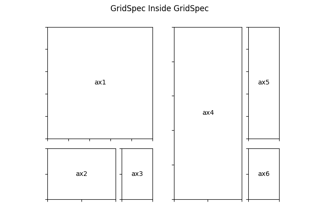Nested Gridspecs

Nested Gridspecs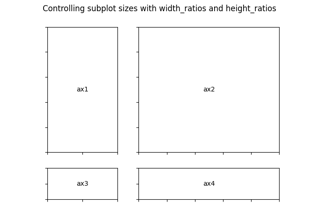GridSpec demo

GridSpec demo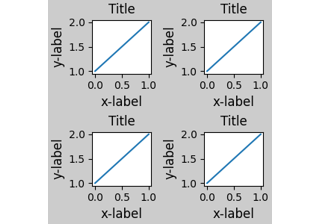Constrained Layout Guide

Constrained Layout Guide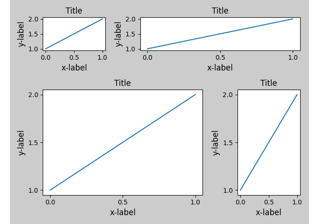Tight Layout guide

Tight Layout guide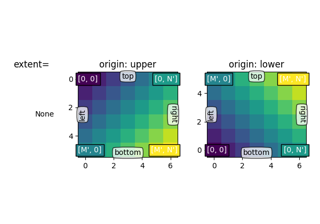origin and extent in imshow

*origin* and *extent* in `~.Axes.imshow`